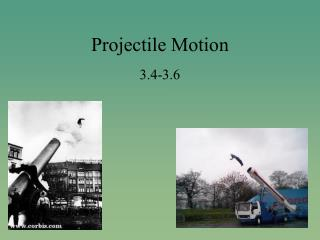Download PresentationProjectile Motion

# Projectile Motion - PowerPoint PPT PresentationDownload Presentation## Projectile Motion

- - - - - - - - - - - - - - - - - - - - - - - - - - - E N D - - - - - - - - - - - - - - - - - - - - - - - - - - -
##### Presentation Transcript

1. Projectile Motion 3.4-3.6

2. Objectives • Describe a projectile • Describe changes of horizontal and vertical components of velocity • Explain why a projectile moves equal distances horizontally in equal time intervals

3. Gravity • How does gravity affect you? • Gravity is all “downwardness.” • There is no “sidewayness” to it. • Shoot and drop dart at same time. Which will hit the ground first? • Gravity does NOT take a holiday on moving objects.

4. Projectiles • What is a projectile? • Projectile: • Object that moves through space acted upon by gravity

5. Projectile Motion • Interactive Figure 10-4 & 10-5 (cd-rom) • There are two components of motion happening in this picture. What are they? • Vertical and Horizontal • Do they affect one another? • No! • Horizontal component of motion is independent of vertical component • What else do you notice? • Horizontal component remains constant • Both balls fall the same vertical distance in the same time (just like darts)

6. The rate of falling is NOT influenced by the horizontal motion • Horizontal component remains the same! • Let's check it out Paul Hewitt Demo!! (cd-rom:10-4, 10-5)

7. Horizontal vs. Vertical What do you notice?? What is the acceleration of the ball falling vertically?

8. The ball is in free fall vertically and moves at a constant speed horizontally (check out the grid lines: horizontal vs. vertical) What do you notice? • Projectile Motion: • Simultaneous horizontal and vertical motions under the influence of gravity.

9. Projectile Motion Notice the shape of path! • What is the name of this shape? • Parabola: • The path traced by a projectile accelerating vertically and maintaining a constant horizontal velocity.

10. Check Question • If I had a slingshot and wanted to hit the door handle in the back of the room, should I aim above it, below it, or directly at it? • Directly at it. • Now, suppose that it takes the rock 1 second to reach a target. Will it fall beneath it? • Yes! How far (if the floor wasn’t in the way)? Hint: d = 1/2gt2 • 5 m • How far above should I aim to hit the target?

11. Upwardly Launched Projectiles • Notice the vertical distances fallen. • d = 1/2gt2 • This is the same physics as free fall only it’s “stretched” out horizontally

12. Check Question • If the cannon were aimed downward instead of upward in the last figure, how would the distances below the new “dashed line” compare?

13. The projectile displacements below the dashed line would be no different. 5m at the end of the 1st second, 20 m at the end of the 2nd second, and so on. Feed the falling monkey!

14. Check Question • Suppose you were flying in a plane that was traveling at 100 m/s and jumped out. Trace the path you would create after 3 seconds?

15. Check Question • You are so frustrated with physics that decide to throw your text book off of the Golden Gate bridge. The speed with which the book leaves your hands is 30 m/s. • Draw the parabolic path that this projectile makes (remember, no air resistance). • How far will it have traveled horizontally AND vertically in 5 seconds? • Hint: d = 1/2gt2

16. Checkpoint 5m • The boy on the tower throws the ball a distance of 20m. At what speed is the ball thrown? • Equation for speed? • v = d/t • What are we missing? • Time! How do we get time ? How long does it take an object to fall 5m? You know this… • 1 sec • This means the ball travels a distance of 20m in 1 second --> velocity of ball is 20m/s 20m

17. Vectors • Vectors can be used to represent the horizontal and vertical components of velocity. • What do you notice about the horizontal and vertical components? (check out Hewit’s cd-rom, 10-9) • Let's check it out!

18. Launching at Different Angles • All projectiles have same initial speed, but different launch angles • What do you notice (look at the numbers . . .)? • The same range is obtained for two angles that add up to 90 degrees! • Animation • To direct water to flowers that are the farthest away, at what angle should a water hose be held? • Maximum range is attained by a 45 degree angle!

19. Satellites • If superman were to throw a ball so fast that it clear the horizon, the ball would then orbit the earth and become an earth satellite. True or False?

20. Satellites • Ball thrown falls 5 m after 1 second (d = 1/2gt2) • If thrown twice as fast, goes twice as far in same time. • 3 times as fast, etc. • The ball’s path curves, so does surface of earth • If ball thrown fast enough to match the curve of earth, it would orbit earth…and become a satellite.

21. Satellite: • a projectile falling fast enough to fall around the earth rather than into it! • 8 km/s or 18,000 mph • Paul Hewitt explains…